Foxtable(狐表)用户栏目专家坐堂 → 查询过于复杂

共有356人关注过本帖树形打印复制链接

# 主题：查询过于复杂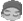wangglby
1楼 | 信息 | 搜索 | 邮箱 | 主页 | UC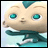加好友发短信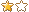以下代码提示查询过于复杂，请问怎么修正？   Dim s11 As StringDim lst1 As new List(of String)Dim filter2 As String = "1=2"With Forms("核价记录").Controls("textbox1")    For i As Integer = 0 To .Lines.Length - 1        s11 = .Lines(i)        lst1.add(s11)    NextEnd With If s11 IsNot Nothing  Then            For Each s12 As String In lst1            filter2 = filter2 & " or 物料信息_名称 like '%" & s12 & "%'"        Next              Dim filter1 As String = "物料信息_物料编码 in ('" & String.join("','",lst1) & "')"        Dim dra As List(Of  DataRow) = DataTables("表A1").SQLSelect( filter2)        Dim lst As new List(of String)         If dra.Count > 0 Then                   For i  As Integer = 0 To dra.Count-1                Dim a As String =dra(i)("物料信息_层级")                Dim c As String =dra(i)("物料信息_名称")                Dim b As String                If a.contains(".") Then   ' 如果 第二列包含" ."                    b = left(a,a.IndexOf("."))    '取第一个" ." 左侧的字符                Else b = a                End If                                If e.form.controls("CheckBox3").checked =False Then                    lst.add(c)                Else                    lst.add(b)                                    End If                            Next        End If        Dim s1() As String = lst.ToArray              Dim filter As String = "1=2"               If e.form.controls("CheckBox3").checked =False Then            For Each s As String In s1                filter = filter & " or 物料信息_名称 like '" & s & "%'"            Next        Else            For Each s As String In s1                filter = filter & " or 物料信息_层级 like '" & s & "%'"            Next        End If        DataTables("表a1").loadFilter = filter          DataTables("表a1").load          End If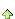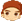2楼 | 信息 | 搜索 | 邮箱 | 主页 | UC加好友发短信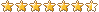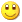Post By：2022/1/20 8:33:00 [只看该作者]

 msgbox(filter)显示什么内容？wangglby
3楼 | 信息 | 搜索 | 邮箱 | 主页 | UC加好友发短信Post By：2022/1/20 9:09:00 [只看该作者]

 我输入“板” 进行查询，filter 如下  估计还没显示全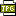此主题相关图片如下：12.jpg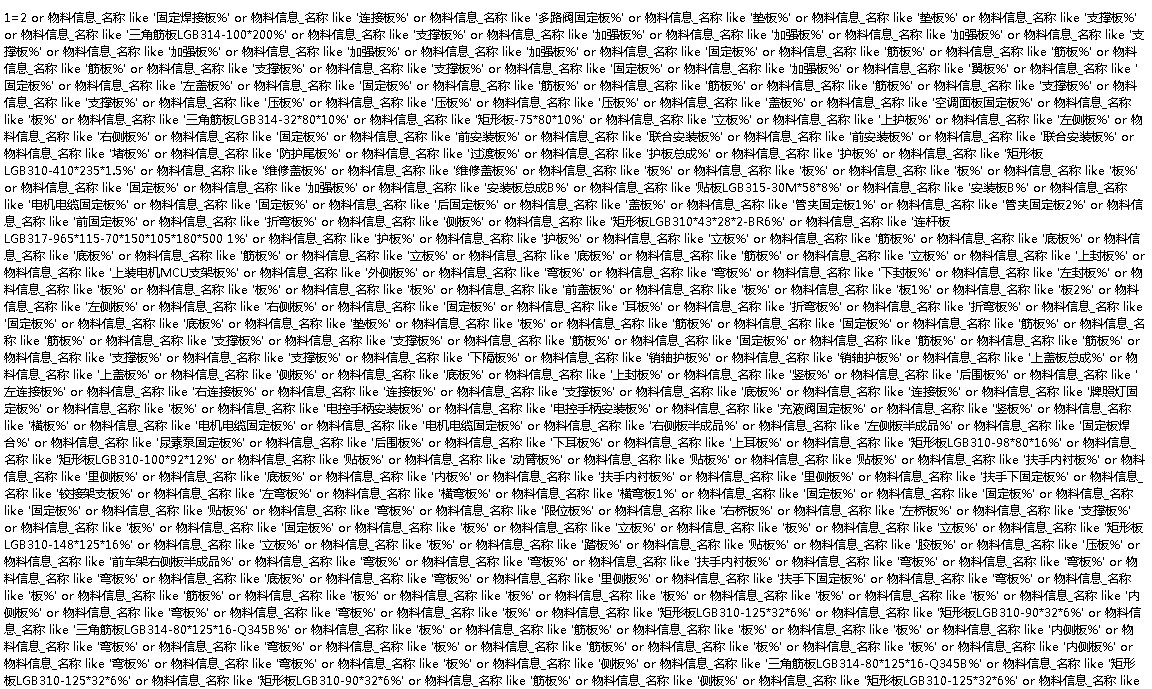4楼 | 信息 | 搜索 | 邮箱 | 主页 | UC加好友发短信Post By：2022/1/20 9:25:00 [只看该作者]

 查询条件是有长度限制的。举例说明一下吧，贴出表数据，说明一下要做怎么样的条件查询